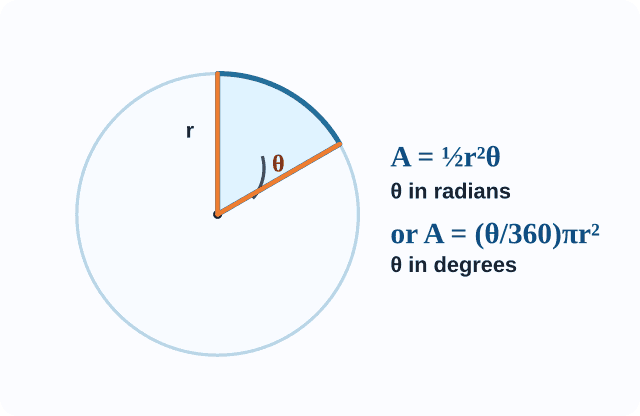# What is the formula to calculate the Area to a sector?

area of segment - area of triangle

( area of eqiulateral triangle is root3/ 4 side2

area of isoceles triangle 1/2 xbase x height [ find base and height through trignometric tables]

area of right triangle )

• 2

Q/360*pie(R)2

WHERE Q=ANGLE MADE BY SECTOR  .

• 2

area of sector is Q/360*pie(R)2... the above is area of segment..

• 1r2Q/360

(where Q=central angle)

• 0

A(sector)=Q/360*pie (r)  or  1/2 *  l*  r  where  l  is length .

• 0

0pi r2/1800

• 0

the area of a sector of angle A= A/ 360 * pie r2

where A = angle in degrees

and pie = 22/7 or 3.14 (as given in the question. If the value of pie is not mentioned , use pie= 22/7.

• 0here is the formula

• 0

Proof:

we know that, Area of a circle = pi x r2

i.e., Area of a sector of angle 3600 = pi x r2

-> Area of a sector of angle 10 = pi x r2 / 360

-> Area of a sector of agle ѳ0 = ѳ/360 x pi x r2 square units

• 1
What are you looking for?